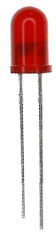﻿ What is the Forward Current, If, of an LED?# What is the Forward Current, IF, of an LED?The forward current of an LED, IF, is the current which flows across the LED's leads, from anode to cathode, in order for the LED to receive sufficient current to power on.As you can see above, positive voltage must be applied across the LED from its anode to its cathode. This leads to a forward current across the LED leads from anode to cathode. If the voltage is reversed, reverse (not forward) current will be produced, and the LED will not light up.

Different kinds of LEDs have different forward current requirements. However, for the vast majority, visible-light LEDs (green, yellow, red LEDs) usually should receive a forward current of about 20mA. Infrared LEDs usually require a bit more current, usually about 50mA of current. Be careful not to apply too much current to an LED, or you risk destroying and blowing out the LED. Two specifications are listed on an LED's datasheet which shows the maximum current an LED can receive. This is the peak forward current and the continuous forward current. Never apply more current to an LED than these specifications.

In order to produce the correct forward current through an LED, place the positive side of the DC power supply to the anode of the LED and then place a resistor in series with the LED to limit the current. The current should be about 20mA if a visible light LED and 50mA if an infrared LED. Calculate the resistor value you would need by using ohm's law, where I(current)= V/R. V is the supply voltage and R is the resistor value you use in the circuit. From there, you should be able to calculate if the current is right for the LED.